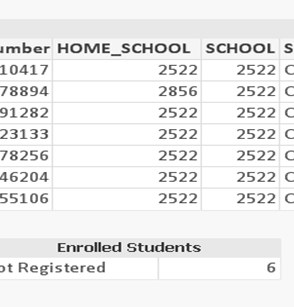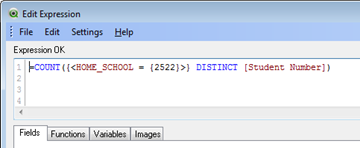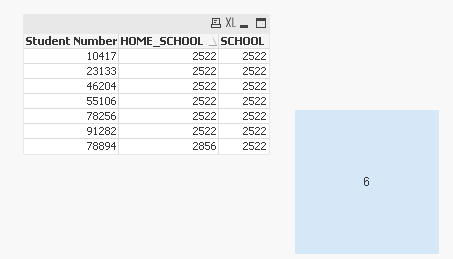# New to QlikView

Discussion board where members can get started with QlikView.

HighlightedNot applicable

## Count in Expression

Hi,

I need to count students with school = home_school only.

Statement : =COUNT({<SCHOOL = HOME_SCHOOL>} DISTINCT [STUDENT NUMBER])

does not give any results.

If I hard code it worksThanks,

L

Tags (1)
1 Solution

Accepted SolutionsMVP

## Re: Count in Expression

Can you try this may be:

=COUNT({<[STUDENT NUMBER] = {"=SCHOOL = HOME_SCHOOL"}>} DISTINCT [STUDENT NUMBER])

18 RepliesMVP

## Re: Count in Expression

Can you try this may be:

=COUNT({<[STUDENT NUMBER] = {"=SCHOOL = HOME_SCHOOL"}>} DISTINCT [STUDENT NUMBER])Not applicable

## Re: Count in Expression

Hi Sunny,

your statement returns the same result as COUNT(DISTINCT [Student Number]), means it doesn't work.

This one works:   =COUNT(DISTINCT if (HOME_SCHOOL = SCHOOL,[Student Number]))

What are the rules? Where to learn the syntax from?

Thanks,MVP

## Re: Count in Expression

try this

=COUNT({<SCHOOL = {"=SCHOOL = HOME_SCHOOL"}>} DISTINCT [STUDENT NUMBER])Not applicable

## Re: Count in Expression

doesn't work.

This one works:   =COUNT(DISTINCT if (HOME_SCHOOL = SCHOOL,[Student Number]))

thanksMVP

## Re: Count in Expression

Although, I am not going to force you to reconsider, but just so you know the if statement is not performance friendly. The reason the above expression might not have worked for you is because you might have copy pasted my expression. Your field name is Student Number and not STUDENT NUMBER (which you gave in your initial post). But if you try this, it might work for you.

=COUNT({<[Student Number]= {"=SCHOOL = HOME_SCHOOL"}>} DISTINCT [Student Number])

QlikView is case sensitive and those two fields are read and understood differently by qlikview.

I am attaching a sample for you to look at.Best,

SunnyNot applicable

## Re: Count in Expression

The prev one didn't work because it is not the same and I did change STUDENT NUMBER to Student Number.

Old: =COUNT({<SCHOOL = {"=SCHOOL = HOME_SCHOOL"}>} DISTINCT [STUDENT NUMBER])

New: =COUNT({<[Student Number]= {"=SCHOOL = HOME_SCHOOL"}>} DISTINCT [Student Number])

Thanks again!!!Not applicable

## Re: Count in Expression

=COUNT({<[Student Number]= {"=SCHOOL = HOME_SCHOOL"}>} DISTINCT [Student Number])

Thanks,

LMVP

## Re: Count in Expression

I did have Student NumberBut that is beside the point.

In my expression, I am checking for each Student Number only include those where School = Home_School. It is like a search string on the Student Number field. The key here is that the field you are searching on should be unique for the search you are trying to do. If for a reason you have 2 student numbers where once it matches and other time it doesn't , then the above expression won't work. Example for where it won't work

Student Number, SCHOOL, HOME_SCHOOL

10, 2522, 2522

10, 2865, 2522

Above we see same Student Number associated with two combination. This won't work now.

Does this make senseNot applicable

## Re: Count in Expression

Thanks again,

It does make sense and it wasn't your statement I was referring to.

Where to learn the syntax from?